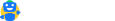File Tax Returns

### Expert assistance on your annual filings

Get Expert Assistance

# Internal Rate of Return – Meaning, Formula and Usage

Updated on:

08 min read

Capital budgeting is a function of management, which uses various techniques to assist in decision making. Internal Rate of Return (IRR) is one such technique of capital budgeting. It is the rate of return at which the net present value of a project becomes zero. They call it ‘internal’ because it does not take any external factor (like inflation) into consideration.

## Introduction

The internal rate of return (IRR) is a discounting cash flow technique which gives a rate of return earned by a project. The internal rate of return is the discounting rate where the total of initial cash outlay and discounted cash inflows are equal to zero. In other words, it is the discounting rate at which the net present value (NPV) is equal to zero.

## How is the Internal Rate of Return computed?

For the computation of the internal rate of return, we use the same formula as NPV. To derive the IRR, an analyst has to rely on trial and error method and cannot use analytical methods. With automation, various software (like Microsoft Excel) is also available to calculate IRR. In Excel, there is a financial function that uses cash flows at regular intervals for calculation.

The rate at which the cost of investment and the present value of future cash flows match will be considered as the ideal rate of return. A project that can achieve this is a profitable project. In other words, at this rate the cash outflows and the present value of inflows are equal, making the project attractive.

## How is IRR used for capital budgeting?

If the same costs apply for different projects, then the project with the highest IRR will be selected. If an organization needs to choose between multiple investment options wherein the cost of investment remains constant, then IRR will be used to rank the projects and select the most profitable one. Ideally, the IRR higher than the cost of capital is selected.

In real life scenarios, since the investment in any project will be huge and will have a long-term effect, an organization uses a combination of various techniques of capital budgeting like NPV, IRR and payback period to select the best project.

Illustration

Let us say a company has an option to replace its machinery.
The cost and return are as follows:
Initial investment = Rs.5,00,000
Incremental increase per year = Rs.2,00,000
Replacement value = Rs.45,270
Life of asset = 3 years
If we assume IRR to be 13%, the computation will be as follows.

 Year Cash flows Discounted cash flows Computation 0 -5,00,000 -500000 (5,00,000 * 1) 1 2,00,000 176991 2,00,000 * (1/1.13)1 2 2,00,000 156229 2,00,000 * (1/1.13)2 3 2,00,000 138610 2,00,000 * (1/1.13)3 4 45,270 27765 45,270 * (1/1.13)4

The total of the column Discounted Cash Flows approximately sums up to zero making the NPV equal to Zero. Hence, this discounted rate is the best rate. As can be seen from the above, using the rate of 13%, the cash flows, both positive and negative become minimum.

Hence, it is the best rate of return on investment. The cost of capital of the company is 10%. Since the IRR is higher than the cost of capital, the project can be selected.

If the company has another opportunity to invest the money in a project that gives a 12% return, the company will still go in for the machinery replacement since it gives the highest IRR.

## What are the shortcomings of the method?

As mentioned earlier, IRR is widely used and adopted by many companies in combination with other techniques for capital budgeting. However, this method has some shortcomings.

• IRR does not take into consideration the duration of the project.
Example, if the company has to choose between two projects – Project A with IRR 15% and duration is one year and Project B with IRR 20% and project duration is 5 years and the cost of capital of the company is 10% – both the projects are profitable. If the company selects Project B because it has a higher IRR it would be incorrect as the duration of Project B is longer.
• IRR assumes that the cash flows are reinvested at the same rate as the project, instead of the cost of capital. Hence, IRR may not give a true picture of the profitability.

Given the shortcomings of the method, analysts are using the Modified Internal Rate of Return. It assumes that the positive cash flows are reinvested at the cost of capital and not IRR.

IRR and NPV together can help one understand the profitability of the project and also choose the most suitable project with a positive NPV. Further, users can compare the IRR of different projects and go for the most profitable one.

#### Related ArticlesFile your income tax for FREE in 7 minutes
Free, simple and accurate. Designed by tax expertsHave a query?
mailto@cleartax.in
Company
Individuals
Tax Experts
SMEs
Enterprises
ITR Resources
MF Resources
Tools
ClearGST
ClearServices